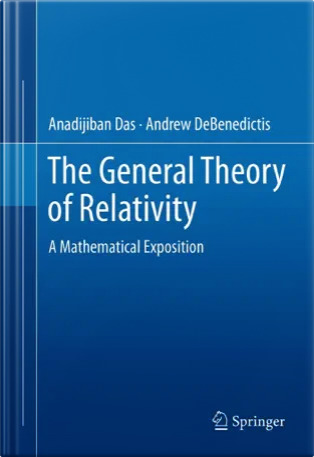The General Theory of Relativity: A Mathematical Exposition

### The General Theory of Relativity: A Mathematical Exposition

Anadijiban Das    &    Andrew DeBenedictisTable of contents,  List of figures,  List of tables,  Index (page numbers correspond to draft version).

Excerpt from the back cover:
This book will serve readers as a modern mathematical introduction to the general theory of relativity. Throughout the book, examples, worked out problems, and exercises (with hints and solutions) are furnished. Topics in this book include, but are not limited to:

 tensor analysis  the special theory of relativity    the general theory of relativity and Einstein's field equations    spherically symmetric solutions and experimental confirmations    static and stationary space-time domains black holes  cosmological models  algebraic classifications and the Newman - Penrose equations  the coupled Einstein-Maxwell-Klein-Gordon equations  appendices covering mathematical supplements and special topics

Mathematical rigor, yet very clear presentation of the topics make this book a unique text for both university students and research scholars.

 Publisher: Springer Number of pages: 710 (approx.) Number of figures: 105 Number of tables: 5 ISBN-10: 1461436575 ISBN-13: 978-1461436577 Publication Date: 2012 Intended audience: Researchers and Graduate students (also appropriate for advanced undergraduates) Publisher's book web page.